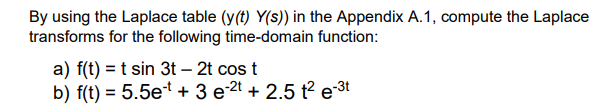# Question By using the Laplace table (y(t) Y(s) in the Appendix A.1, compute the Laplace transforms for the following time-domain function: a) f(t) = t sin 3t - 2t cost b) f(t) = 5.5e-* + 3 e 2 + 2.5 t e-3tT5F3DH The Asker · Mechanical EngineeringTranscribed Image Text: By using the Laplace table (y(t) Y(s) in the Appendix A.1, compute the Laplace transforms for the following time-domain function: a) f(t) = t sin 3t - 2t cost b) f(t) = 5.5e-* + 3 e 2 + 2.5 t e-3t
More
Transcribed Image Text: By using the Laplace table (y(t) Y(s) in the Appendix A.1, compute the Laplace transforms for the following time-domain function: a) f(t) = t sin 3t - 2t cost b) f(t) = 5.5e-* + 3 e 2 + 2.5 t e-3t